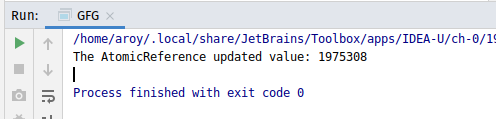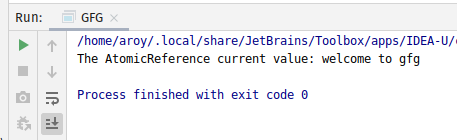# AtomicReference updateAndGet() method in Java with Examples

The updateAndGet() method of a AtomicReference class is used to atomically updates which updates the current value of the AtomicReference by applying the specified updateFunction operation on the current value. It takes an object of updateFunction interface as its parameter and applies the operation specified in the object to the current value. It returns the updated value.

Syntax:

```public final V
updateAndGet(UnaryOperator<V> updateFunction)
```

Parameters: This method accepts updateFunction which is a side-effect-free function.

Return value: This method returns the updated value.

Below programs illustrate the updateAndGet() method:
Program 1:

 `// Java program to demonstrate ` `// AtomicReference.updateAndGet() method ` ` `  `import` `java.util.concurrent.atomic.*; ` `import` `java.util.function.UnaryOperator; ` ` `  `public` `class` `GFG { ` `    ``public` `static` `void` `main(String args[]) ` `    ``{ ` ` `  `        ``// AtomicReference with value ` `        ``AtomicReference ref ` `            ``= ``new` `AtomicReference<>(``987654``); ` ` `  `        ``// Declaring the updateFunction ` `        ``// applying function ` `        ``UnaryOperator function ` `            ``= (v) -> Integer.parseInt(v.toString()) * ``2``; ` ` `  `        ``// apply updateAndGet() ` `        ``int` `value = ref.updateAndGet(function); ` ` `  `        ``// print AtomicReference ` `        ``System.out.println( ` `            ``"The AtomicReference updated value: "` `            ``+ value); ` `    ``} ` `} `

Output:Program 2:

 `// Java program to demonstrate ` `// AtomicReference.updateAndGet() method ` ` `  `import` `java.util.concurrent.atomic.*; ` `import` `java.util.function.UnaryOperator; ` ` `  `public` `class` `GFG { ` `    ``public` `static` `void` `main(String args[]) ` `    ``{ ` ` `  `        ``// AtomicReference with value ` `        ``AtomicReference ref ` `            ``= ``new` `AtomicReference<>(``"welcome"``); ` ` `  `        ``// Declaring the updateFunction ` `        ``// applying function to add value as string ` `        ``UnaryOperator twoDigits ` `            ``= (v) -> v + ``" to gfg"``; ` ` `  `        ``// apply updateAndGet() ` `        ``String value ` `            ``= ref.updateAndGet(twoDigits); ` ` `  `        ``// print AtomicReference ` `        ``System.out.println( ` `            ``"The AtomicReference current value: "` `            ``+ value); ` `    ``} ` `} `

Output:Whether you're preparing for your first job interview or aiming to upskill in this ever-evolving tech landscape, GeeksforGeeks Courses are your key to success. We provide top-quality content at affordable prices, all geared towards accelerating your growth in a time-bound manner. Join the millions we've already empowered, and we're here to do the same for you. Don't miss out - check it out now!

Previous
Next